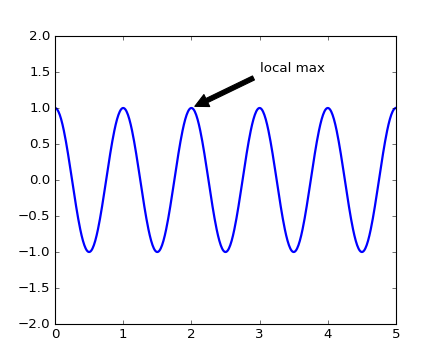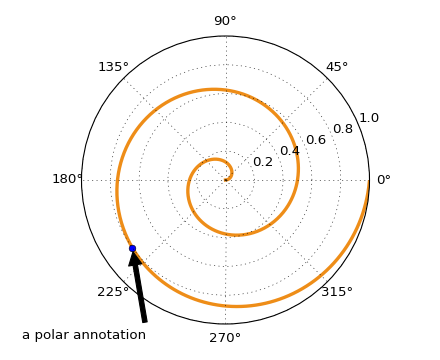## Matplotlib 2.0.0rc2 is available

Install the release candidate now!Travis-CI:# Annotating text¶

For a more detailed introduction to annotations, see Annotating Axes.

The uses of the basic `text()` command above place text at an arbitrary position on the Axes. A common use case of text is to annotate some feature of the plot, and the `annotate()` method provides helper functionality to make annotations easy. In an annotation, there are two points to consider: the location being annotated represented by the argument `xy` and the location of the text `xytext`. Both of these arguments are `(x,y)` tuples.

```import numpy as np
import matplotlib.pyplot as plt

fig = plt.figure()

t = np.arange(0.0, 5.0, 0.01)
s = np.cos(2*np.pi*t)
line, = ax.plot(t, s, lw=2)

ax.annotate('local max', xy=(2, 1), xytext=(3, 1.5),
arrowprops=dict(facecolor='black', shrink=0.05),
)

ax.set_ylim(-2,2)
plt.show()
```In this example, both the `xy` (arrow tip) and `xytext` locations (text location) are in data coordinates. There are a variety of other coordinate systems one can choose – you can specify the coordinate system of `xy` and `xytext` with one of the following strings for `xycoords` and `textcoords` (default is ‘data’)

argument coordinate system
‘figure points’ points from the lower left corner of the figure
‘figure pixels’ pixels from the lower left corner of the figure
‘figure fraction’ 0,0 is lower left of figure and 1,1 is upper right
‘axes points’ points from lower left corner of axes
‘axes pixels’ pixels from lower left corner of axes
‘axes fraction’ 0,0 is lower left of axes and 1,1 is upper right
‘data’ use the axes data coordinate system

For example to place the text coordinates in fractional axes coordinates, one could do:

```ax.annotate('local max', xy=(3, 1),  xycoords='data',
xytext=(0.8, 0.95), textcoords='axes fraction',
arrowprops=dict(facecolor='black', shrink=0.05),
horizontalalignment='right', verticalalignment='top',
)
```

For physical coordinate systems (points or pixels) the origin is the (bottom, left) of the figure or axes. If the value is negative, however, the origin is from the (right, top) of the figure or axes, analogous to negative indexing of sequences.

Optionally, you can specify arrow properties which draws an arrow from the text to the annotated point by giving a dictionary of arrow properties in the optional keyword argument `arrowprops`.

`arrowprops` key description
width the width of the arrow in points
frac the fraction of the arrow length occupied by the head
headwidth the width of the base of the arrow head in points
shrink move the tip and base some percent away from the annotated point and text
**kwargs any key for `matplotlib.patches.Polygon`, e.g., `facecolor`

In the example below, the `xy` point is in native coordinates (`xycoords` defaults to ‘data’). For a polar axes, this is in (theta, radius) space. The text in this example is placed in the fractional figure coordinate system. `matplotlib.text.Text` keyword args like `horizontalalignment`, `verticalalignment` and ```fontsize are passed from the `~matplotlib.Axes.annotate` to the ``Text``` instance

```import numpy as np
import matplotlib.pyplot as plt

fig = plt.figure()
r = np.arange(0,1,0.001)
theta = 2*2*np.pi*r
line, = ax.plot(theta, r, color='#ee8d18', lw=3)

ind = 800
thisr, thistheta = r[ind], theta[ind]
ax.plot([thistheta], [thisr], 'o')
ax.annotate('a polar annotation',